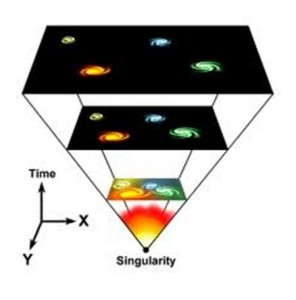# What is Physics?

Physics involves the study of nature in order to understand how the universe behaves.

It involves analysis of the behavior of the small subatomic particles to the large cluster of galaxies.One may wonder about a lot of things like:

• Why is the sky blue?
• Why does fire give out heat?
• How do birds fly?
• How do magnets attract or repel another magnet?

And plenty of other questions!

We try and understand why such things happen in this subject.

Physics essentially as we will slowly understand, is the study of matter, energy and how these interact.

The whole universe is composed of different forms of matter and energy and their interaction results in all the various phenomena that we observe.

## Matter

Matter or mass is everything that occupies space. In layman’s terms matter or mass is everything that we can touch. From electrons, protons to items such as wood, steel, water and the sun, moon, etc. Everything is made of matter or mass

Mass is essentially of two types:

• Inertial mass
• Gravitational mass

Inertial mass indicates how hard it is to accelerate an object when you apply force on it.

Gravitational mass gives an idea about how large the gravitational force the object experiences.

Mass of an object gives it many interesting characteristics! Planets in the solar system revolve around the sun because of the gravitational interaction between them due to their masses.

## Energy

Energy is the ability to do work.

Work is the process of moving an object

Thus if we put the above two definitions together, energy is basically the ability to move an object. Energy can be in various forms such as heat, sound, light, etc. And each of these different forms of energy at some microscopic or macroscopic level is movement of some objects due to various physical reasons.

For example if move an object, it will have kinetic energy. If we then move it very fast and smash it against something, the kinetic energy can get transformed into heat and sound energy.Energy can be changed from one form to another but cannot be ever created out of nothing, nor can it be destroyed into nothingness.

## Mass Energy Equivalence

Einstein, one of the most celebrated physicist of all time figured out that mass and energy can be converted into each other. Thus mass is also a form of energy.

He formulated the equation E=mc² , which governs the conversion mass into energy and vice versa. ‘c’ in this equation represents speed of light.

## Study of Everything

Thus if physics is the study of mass and energy, and almost everything in this universe is made up of mass and energy, physics effectively becomes the study of everything.

That seems like a huge task! So as of now we will be restricted to a few topics of interest. Such as :

• Mechanics: This involves studying mechanical interactions such as how velocity and gravitational forces of objects affect different phenomena in the macroscopic world.
• Fluids: This deals with fluid materials such as water and gas.
• Thermal physics: This topic deals with heat energy and work done.
• Electricity and magnetism: This topic deals with objects which are affected by electric or magnetic fields. The concept of electricity, circuits, etc are also dealt with under this topic.
• Waves and optics: This topic deals with light, its path, its interactions with objects, etc.
• Modern physics: Modern physics essentially deals with the topics that have been developed over the last century, such as nuclear physics, relativity, etc.

[box type=”success” align=”” class=”” width=””]For a more elegant explanation of what is physics you can check out the lecture : What is physics?[/box]

SPECIAL OFFER!

`SUCCESS8`